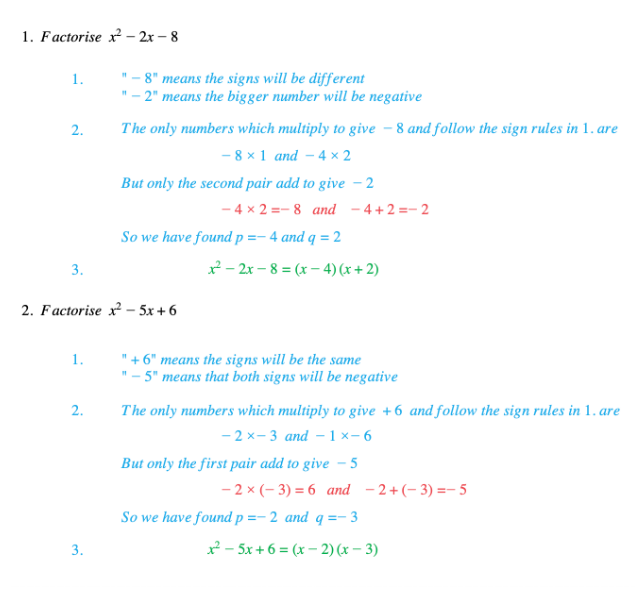# Edexcel IGCSE Maths 复习笔记 2.4.1 Factorising Quadratics - Basics

Edexcel IGCSE Maths 复习笔记 2.4.1 Factorising Quadratics - Basics

#### What is a quadratic expression?

• A quadratic expression looks like this:ax2 + bx + c (as long as a ≠ 0)
• Note: If there are any higher powers of x (like x3 say) then it is not a quadratic!

#### Factorising a 3-term quadratic expression (a = 1)

• Signs in quadratic determine signs in brackets:
• if c is positive then both signs are the same as the sign of b
• if c is negative then the signs are different and bigger number has the sign of b

• Using those signs find numbers p and q which
• multiply to give c

• Write down the brackets (x + p)(x + q) – that’s your answer!Don’t forget – both p and q here can be negative!

#### Exam Tip

Make sure you know if you are being asked to:

• Solve an equation (look for the “=”) or
• Factorise an expression (no “=”)

Do not confuse the two things.

When the quadratic expression only has two terms check for:

• Simple factorisation (no number term, ie. when c = 0 )
• Difference Of Two Squares (no x term, ie. when b = 0)

Worked Example